# MineralsPage 6

#### WATCH ALL SLIDES

One Type of Asbestos

5. Silicates are the most important mineral group

Slide 54Tectosilicates - Three-Dimensional Networks

Quartz Feldspars

5. Silicates are the most important mineral group

Slide 55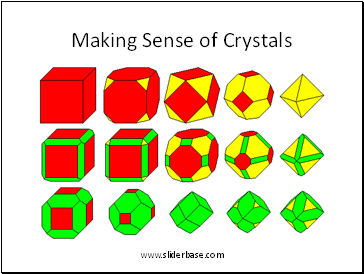Making Sense of Crystals

6. Crystals are determined by mathematical rules called symmetry

Slide 56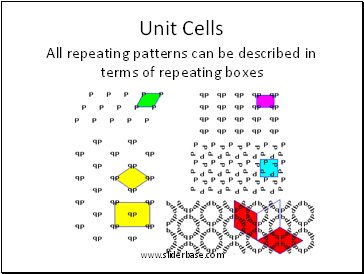## Unit Cells

All repeating patterns can be described in terms of repeating boxes

Slide 57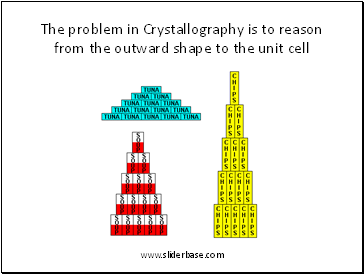The problem in Crystallography is to reason from the outward shape to the unit cell

6. Crystals are determined by mathematical rules called symmetry

Slide 58Which Shape Makes Each Stack?

Crystals are determined by mathematical rules called symmetry

Slide 59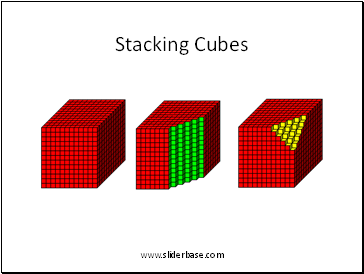Stacking Cubes

6. Crystals are determined by mathematical rules called symmetry

Slide 60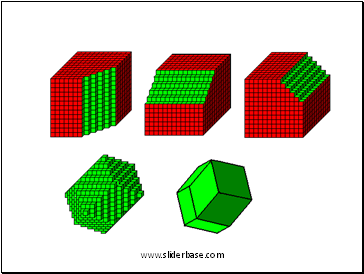6. Crystals are determined by mathematical rules called symmetry

Slide 61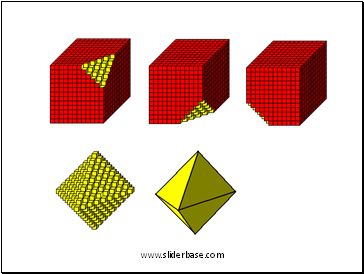6. Crystals are determined by mathematical rules called symmetry

Slide 62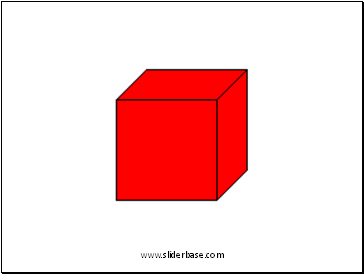6. Crystals are determined by mathematical rules called symmetry

Slide 63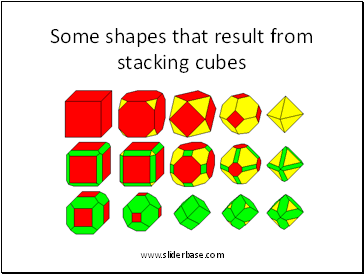Some shapes that result from stacking cubes

6. Crystals are determined by mathematical rules called symmetry

Slide 64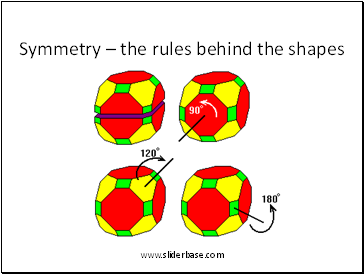Symmetry – the rules behind the shapes

6. Crystals are determined by mathematical rules called symmetry

Slide 65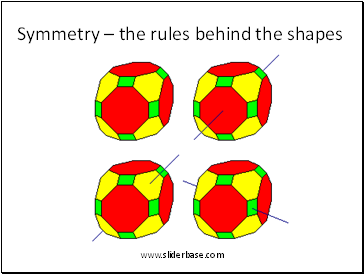Symmetry – the rules behind the shapes

6. Crystals are determined by mathematical rules called symmetry

Slide 66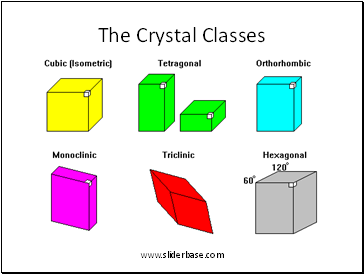The Crystal Classes

6. Crystals are determined by mathematical rules called symmetry

Slide 67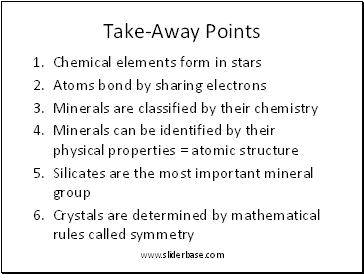Take-Away Points

Chemical elements form in stars

Atoms bond by sharing electrons

Minerals are classified by their chemistry

Minerals can be identified by their physical properties = atomic structure

Go to page:
1  2  3  4  5  6  7